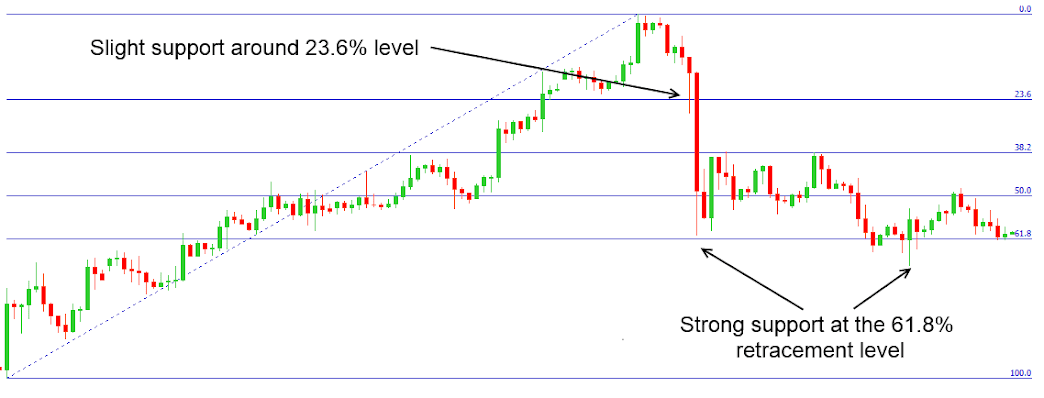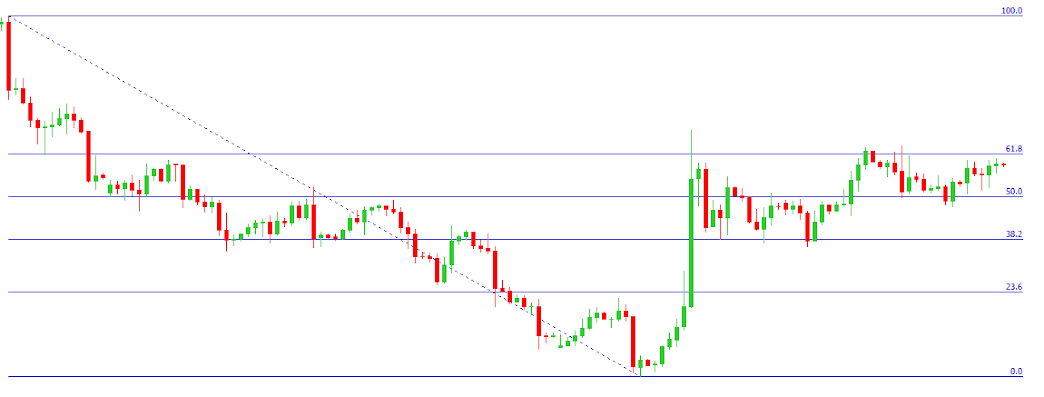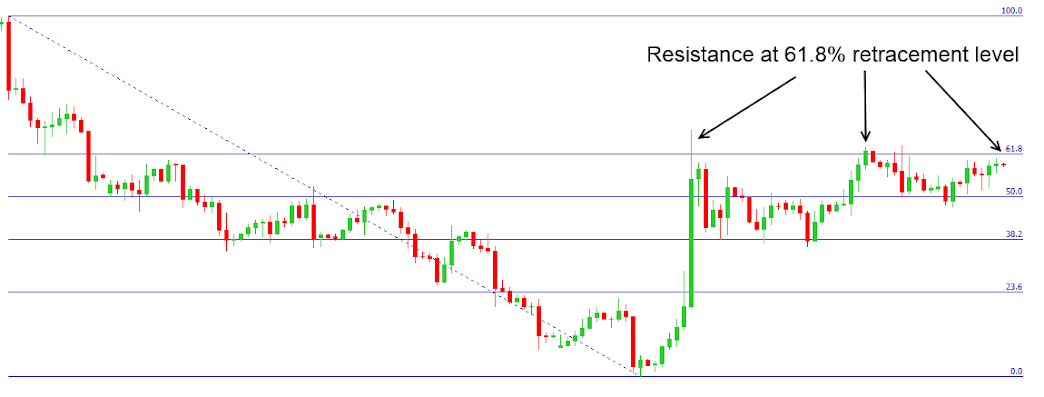Who was Fibonacci?

Leonardo Pisano Bigollo better known as Leonardo Fibonacci was an Italian mathematician. Fibonacci is often considered to have been the most talented mathematician of the middle ages and is best known for a number sequence named after him.  The number sequence is begins with 0 followed by 1 and then continues by adding 0+1 to derive; 1 the third number in the sequence.   The sequence then continues by adding the second and third number together getting 2. The sequence continues to progress in the same way.

• The Fibonacci number sequence: 0, 1, 1, 2, 3, 5, 8, 13, 21, 34, 55, 89, 144….

Fibonacci Ratios

After the first few numbers in sequence, if you measure the ratio of any two succeeding numbers you arrive at the ratio of 0.618. For example if you divide 89 by 144, you arrive at a ratio of 0.618.

Again after the first few numbers in the sequence, if you measure the ratio between two alternate numbers you get a ratio of 0.382. You can try this with the above Fibonacci number sequence; for example if you divide 13 by 32 you arrive at 0.382.

These recurring ratios are known as the golden mean and it has been claimed that these ratios naturally occur in nature. Traders have made use of these Fibonacci ratios to create a number of Fibonacci studies, using a series of retracement and extension levels.

Need To Know Fibonacci Retracement and Extension Levels

• Retracement Levels: 0.236, 0.382, 0.500, 0.618, 0.764
• Extension Levels:  0, 0.382, 0.618, 1.00, 1.382, 1.618

You are probably never going to have calculate these levels yourself, as the overwhelming majority of charting software will do all the work for you. There are also a number of Fibonacci calculators available online.

These retracement and extension levels are used as potential support and resistance levels. It has been argued that Fibonacci levels are a self-fulfilling prophecy, due to the sheer number of traders using Fibonacci levels in their trading strategy.

Using Fibonacci Retracement and Extension Levels

Fibonacci Retracements

It’s important to note that Fibonacci tools are most effectively used when markets are trending. When markets are trending upwards traders go long (Buy) when the price hits a particular Fibonacci retracement. When markets are trending downwards traders go short (Sell) when the market price hits a particular Fibonacci retracement level.

Fibonacci Retracements: Uptrends

To begin you have to draw a line from the start of the trend to the trends high point. If you use the Fibonacci retracement tool on MetaTrader the retracement levels will be automatically placed over the chart.As you can see from the above chart, the retracement levels are set at 23.6%, 38.2%, 50.0% and at 61.8% of the trend. Many traders believe that if the trend begins to retrace from the trend high, the instrument will find support at one of Fibonacci levels. As it is thought that traders will place buy orders around at the various Fibonacci retracement levels.In the above example, you can see that the USD/CHF found brief support at the 23.6% retracement level. Before falling through to find stronger support around the 61.8% retracement level, where presumably traders buy orders kicked in. The instrument recovered to around the 38.2% retracement level before again falling towards the 61.8% retracement level, where the USD/CHF again found strong support.

Fibonacci Retracements: DowntrendsTo begin you have draw a trend line from to the start of the trend to the trend low. Many traders believe that as the downward trend retraces on itself the instrument will find resistance at one of the Fibonacci retracement levels. As traders have placed Sell orders around the various Fibonacci levels.In the above example, you can see that the EUR/USD began to retrace the previous downward trend pretty rapidly. But found significant resistance level around the 61.8% retracement level. Presumably traders have decided to place sell orders around the 61.8% mark, which means the EUR/USD faces significant resistance around the retracement level.

When Fibonacci Levels Fail

The above examples show instruments finding significant resistance around one or more of the Fibonacci retracement levels. The markets have tendency to break through resistance and support levels, often at a cost to those who have placed orders on the basis of Fibonacci studies.

While Fibonacci levels can be a useful tool traders have to realise that they have limitations. You don’t always know at which retracement level the instrument will find support or resistance, meaning that you can place an order only to see the market move against you.

It is for these reasons that Fibonacci studies are often used alongside other technical and charting techniques. Fibonacci levels can be a useful tool in a traders arsenal, though traders have to realise the limitations of the technique.